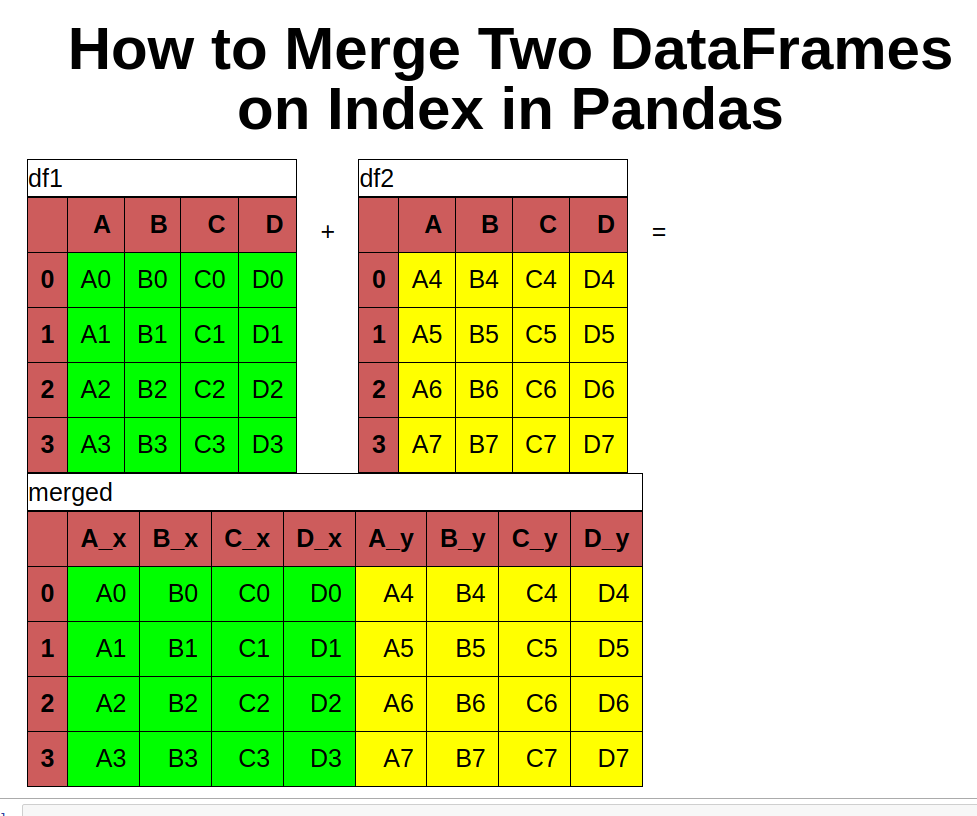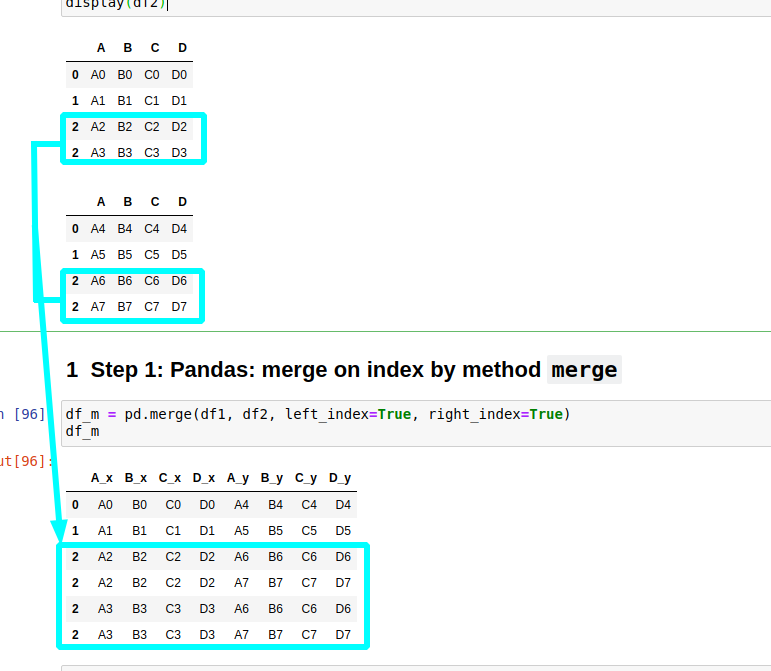In this short tutorial, we'll show how to merge two DataFrames on index in Pandas.

Here are two approaches to merge DataFrames on index:

(1) Use method `merge` with `left_index` and `right_index`

``````pd.merge(df1, df2, left_index=True, right_index=True)
``````

(2) Method `concat` with `axis=1`

``````pd.concat([df1, df2], axis=1)
``````

To start, let's say that we have two DataFrames:

df1

A B C D
A0 B0 C0 D0
A1 B1 C1 D1
A2 B2 C2 D2
A3 B3 C3 D3

df2

A B C D
A4 B4 C4 D4
A5 B5 C5 D5
A6 B6 C6 D6
A7 B7 C7 D7

## Option 1: Pandas: merge on index by method `merge`

The first example will show how to use method `merge` in combination with `left_index` and `right_index`.

This will merge on index by `inner join` - only the rows from the both DataFrames with similar index will be added to the result:

``````pd.merge(df1, df2, left_index=True, right_index=True)
``````

The result is:

A_x B_x C_x D_x A_y B_y C_y D_y
A0 B0 C0 D0 A4 B4 C4 D4
A1 B1 C1 D1 A5 B5 C5 D5
A2 B2 C2 D2 A6 B6 C6 D6
A3 B3 C3 D3 A7 B7 C7 D7As you can see the result DataFrame is a combination of both merged on the index. The column names have suffixes: `_x` and `_y`.

In order to change the suffixes use the following syntax:

``````df_m = pd.merge(df1, df2, left_index=True, right_index=True, suffixes=('_left', '_right'))
df_m
``````

which uses parameter `suffixes: 'Suffixes' = ('_x', '_y')`

The functions is described as:

Merge DataFrame or named Series objects with a database-style join.

### Duplicated index while merging on index

In case of duplicated index while merging on index the rows will be duplicated:## Option 2: Pandas: merge on index by `concat` and axis=1

Alternative solution is to merge both DataFrames by using method `concat`. For this approach you need to set the parameter `axis=1` which is equivalent to `axis='columns'`.

``````pd.concat([df1, df2], axis='columns')
``````

The result is similar to the previous one with differences in the column names. Using `concat` will not change the column names.

A B C D A B C D
A0 B0 C0 D0 A4 B4 C4 D4
A1 B1 C1 D1 A5 B5 C5 D5
A2 B2 C2 D2 A6 B6 C6 D6
A3 B3 C3 D3 A7 B7 C7 D7

As you can see we have duplicate names for the columns. In order to avoid `concat` with duplicated columns use `verify_integrity`:

``````df_m = pd.concat([df1, df2], axis='columns', verify_integrity=True)
df_m
``````

This will raise error:

ValueError: Indexes have overlapping values: Index(['A', 'B', 'C', 'D'], dtype='object')

If you still prefer to have duplicate columns you can access them in the normal way:

``````df_m['A']
``````

result:

A A
A0 A4
A1 A5
A2 A6
A3 A7

## Option 3: Pandas: merge on index - `join`

Method `join` can be used for the merge operation. By default is `left join`.

In case of duplicated column names will error:

ValueError: columns overlap but no suffix specified: Index(['A', 'B', 'C', 'D'], dtype='object')

In order to avoid errors use the following syntax:

``````df1.join(df2, lsuffix='_x')
``````

## Conclusion: Pandas: merge on index - `merge` vs `concat`

Finally let's compare the both ways:

• `merge` will assign suffixes for both DataFrames / `concat` will not change the column names
• `merge` has control on the merge operation. By default the operation is `inner join` - you can change it to `left`, `right`, `outer` etc
• `merge`

So if we add extra row for df2 as:

A B C D
0 A4 B4 C4 D4
1 A5 B5 C5 D5
2 A6 B6 C6 D6
3 A7 B7 C7 D7
4 A8 B8 C8 D8

Then concat will do full outer join by default:

A B C D A B C D
0 A0 B0 C0 D0 A4 B4 C4 D4
1 A1 B1 C1 D1 A5 B5 C5 D5
2 A2 B2 C2 D2 A6 B6 C6 D6
3 A3 B3 C3 D3 A7 B7 C7 D7
4 NaN NaN NaN NaN A8 B8 C8 D8

While merge will do only inner join and the result will be the same as in Step 1. In order to change `merge` behaviour you need to change the `how` parameter:

``````df_m = pd.merge(df1, df2, left_index=True, right_index=True, how='outer')
df_m
``````

So the The best way to merge on an index is to use the method `merge`.